## Saturday, 9 August 2014

### chapter 6 Some Standard Limits 2

(C)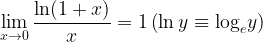$\mathop {\lim }\limits_{x \to 0} \dfrac{{\ln (1 + x)}}{x} = 1\left( {\ln y \equiv {{\log }_e}y} \right)$
This limit is of the indeterminate form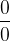$\dfrac{0}{0}$ . We can easily evaluate this limit based on the previous limit.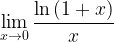$\mathop {\lim }\limits_{x \to 0} \dfrac{{\ln \left( {1 + x} \right)}}{x}$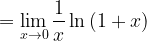$= \mathop {\lim }\limits_{x \to 0} \dfrac{1}{x}\ln \left( {1 + x} \right)$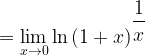$= \mathop {\lim }\limits_{x \to 0} \ln {\left( {1 + x} \right)^{\dfrac{1}{x}}}$ (Property of log)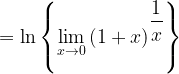$= \ln \left\{ {\mathop {\lim }\limits_{x \to 0} {{\left( {1 + x} \right)}^{\dfrac{1}{x}}}} \right\}$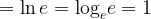$= \ln e = {\log _e}e = 1$
This limit can alternatively be evaluated by using the expansion series for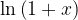$\ln \left( {1 + x} \right)$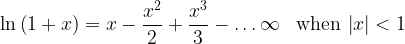$\ln \left( {1 + x} \right) = x - \dfrac{{{x^2}}}{2} + \dfrac{{{x^3}}}{3} - \ldots\infty \,\,\,\,\,{\rm{when}}\,\left| x \right| < 1$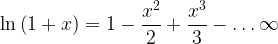$\ln \left( {1 + x} \right) = 1 - \dfrac{{{x^2}}}{2} + \dfrac{{{x^3}}}{3} - \ldots\infty$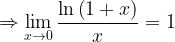$\Rightarrow \mathop {\lim }\limits_{x \to 0} \dfrac{{\ln \left( {1 + x} \right)}}{x} = 1$ (All other terms involving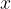$x$ tend to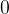$0$
This is just an obvious extension of the previous limit(D)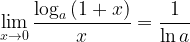$\mathop {\lim }\limits_{x \to 0} \dfrac{{{{\log }_a}\left( {1 + x} \right)}}{x} = \dfrac{1}{{\ln a}}$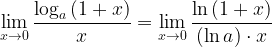$\mathop {\lim }\limits_{x \to 0} \dfrac{{{{\log }_a}\left( {1 + x} \right)}}{x} = \mathop {\lim }\limits_{x \to 0} \dfrac{{\ln \left( {1 + x} \right)}}{{\left( {\ln a} \right) \cdot x}}$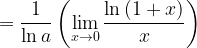$= \dfrac{1}{{\ln a}}\left( {\mathop {\lim }\limits_{x \to 0} \dfrac{{\ln \left( {1 + x} \right)}}{x}} \right)$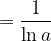$= \dfrac{1}{{\ln a}}$
We have used the following property of logarithms above: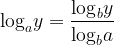${\log _a}y = \dfrac{{{{\log }_b}y}}{{{{\log }_b}a}}$
(E)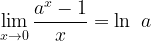${\mathop {\lim }\limits_{x \to 0} \dfrac{{{a^x} - 1}}{x} = \ln \,\,a}$
This is again an extension of the limits seen previously. Let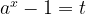${a^x} - 1 = t$. This gives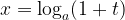$x = {\log _a}(1 + t)$ As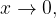$x \to 0,$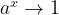${a^x} \to 1$ and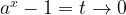${a^x} - 1 = t \to 0$
Hence, we have the limit L as$L = \mathop {\lim }\limits_{x \to 0} \dfrac{{{a^x} - 1}}{x} = \mathop {\lim }\limits_{t \to 0} \dfrac{t}{{{{\log }_a}\left( {1 + t} \right)}}$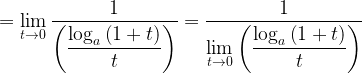$= \mathop {\lim }\limits_{t \to 0} \dfrac{1}{{\left( {\dfrac{{{{\log }_a}\left( {1 + t} \right)}}{t}} \right)}} = \dfrac{1}{{\mathop {\lim }\limits_{t \to 0} \left( {\dfrac{{{{\log }_a}\left( {1 + t} \right)}}{t}} \right)}}$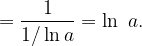$= \dfrac{1}{{1/\ln a}} = \ln \,\,a.$
Note that for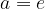$a = e$, this limit is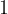$1$.
(F)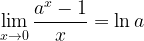$\mathop {\lim }\limits_{x \to 0} \dfrac{{{a^x} - 1}}{x} = \ln a$
When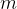$m$ is an integer, it is easy to see that the above relation holds because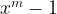${x^m} - 1$ can be expanded as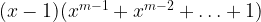$(x - 1)({x^{m - 1}} + {x^{m - 2}} + \ldots + 1)$

Now we have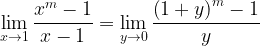$\mathop {\lim }\limits_{x \to 1} \dfrac{{{x^m} - 1}}{{x - 1}} = \mathop {\lim }\limits_{y \to 0} \dfrac{{{{\left( {1 + y} \right)}^m} - 1}}{y}$ . Now expand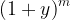${\left( {1 + y} \right)^m}$ using the Binomial theorem for a general index.For the general case, let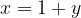$x = 1 + y$. As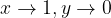$x \to 1,y \to 0$.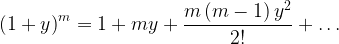${\left( {1 + y} \right)^m} = 1 + my + \dfrac{{m\left( {m - 1} \right){y^2}}}{{2!}} + \ldots$
Hence,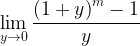$\mathop {\lim }\limits_{y \to 0} \dfrac{{{{\left( {1 + y} \right)}^m} - 1}}{y}$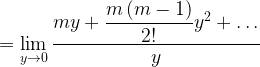$= \mathop {\lim }\limits_{y \to 0} \dfrac{{my + \dfrac{{m\left( {m - 1} \right)}}{{2!}}{y^2} + \ldots}}{y}$$= \mathop {\lim }\limits_{y \to 0} \left( {m + \dfrac{{m\left( {m - 1} \right)}}{{2!}}y + \ldots} \right)$$=m$ (all other terms tend to$0$